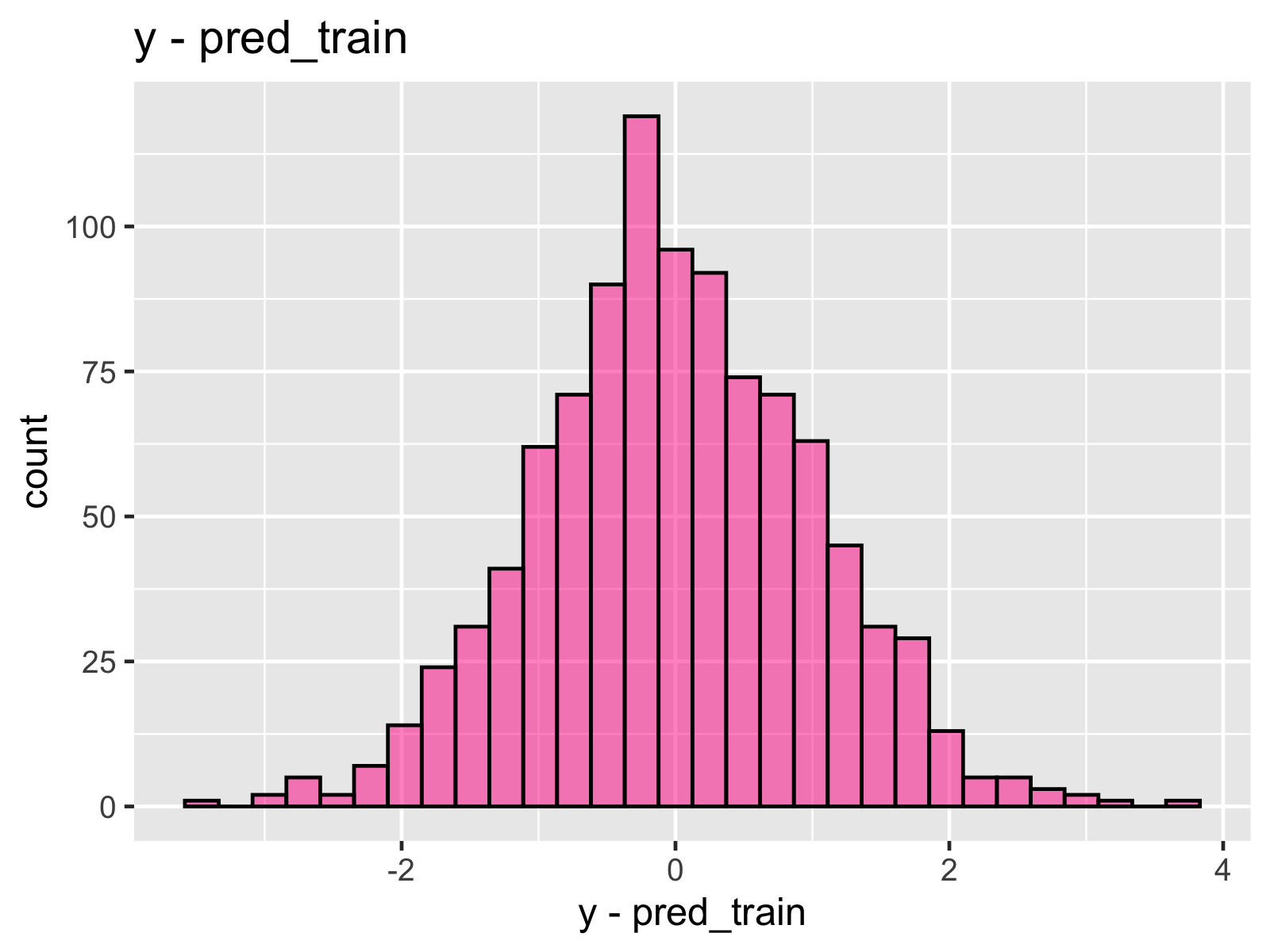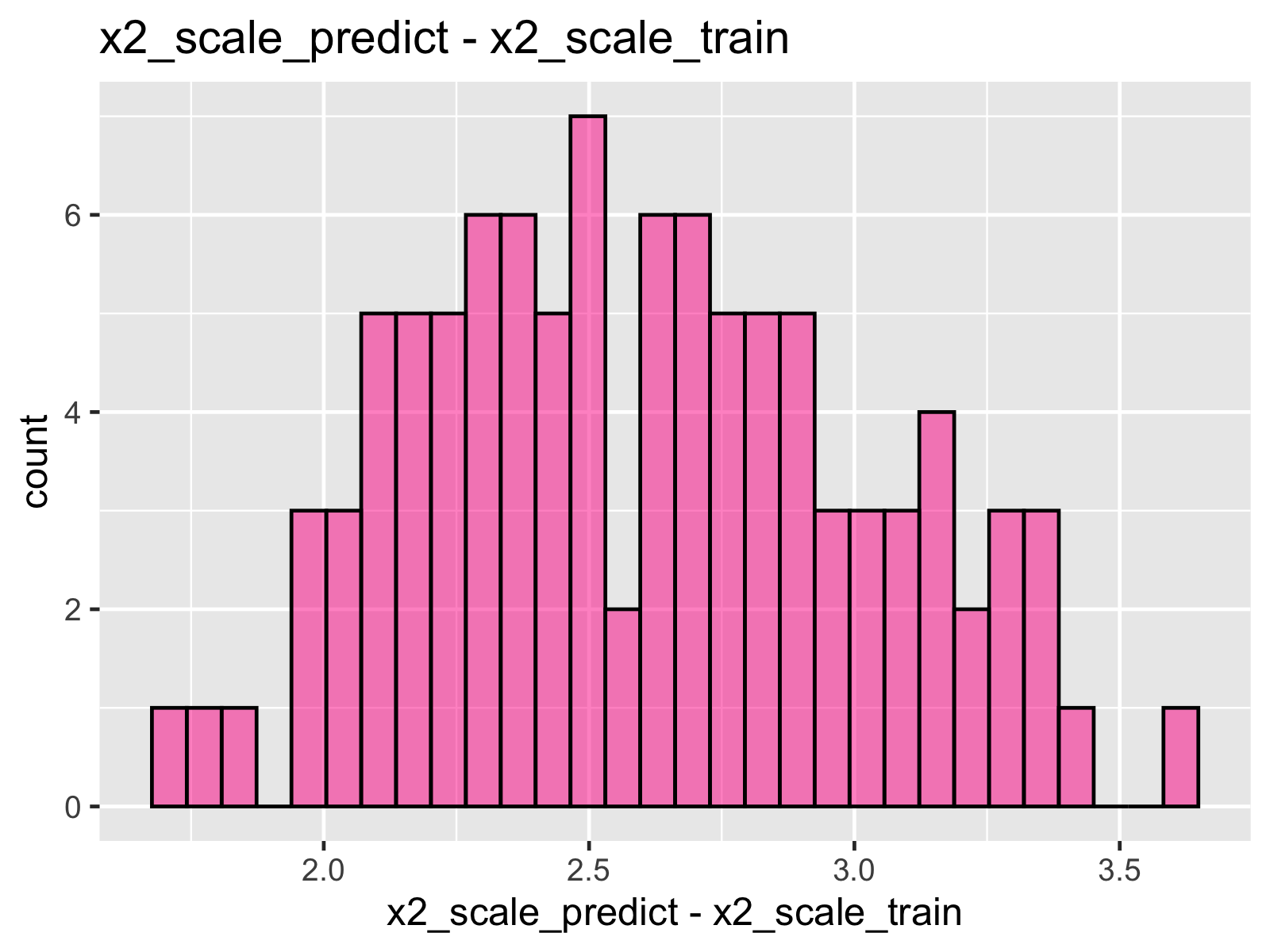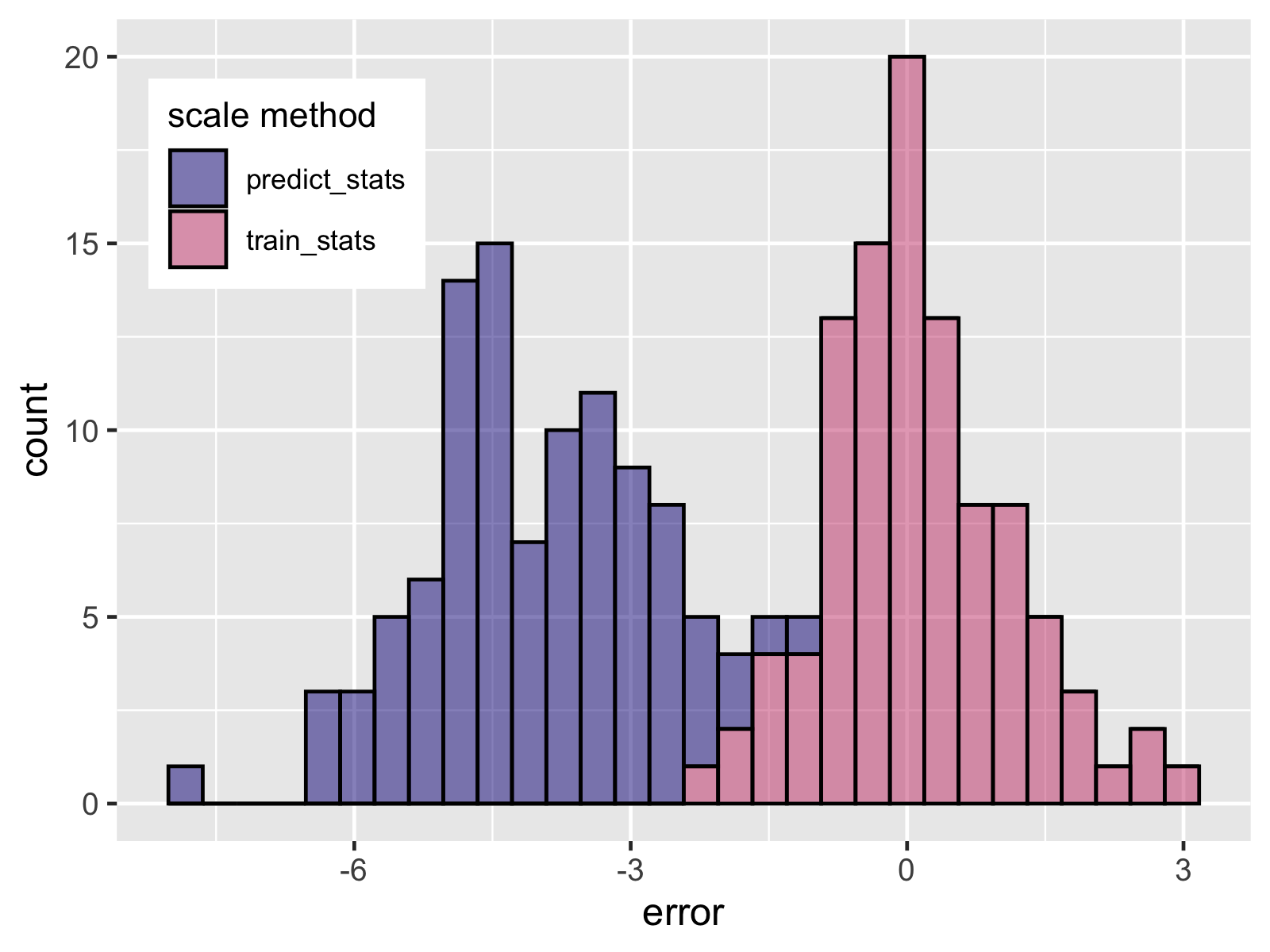This post runs through why it’s important to preprocess new data that you’re passing to a Machine Learning model using statistics calculated from the training data. First off, we’ll build some intuition with a simple example in R. Then we’ll turn to tools like scikit-learn pipelines, which easily allow you to do this the right way.

# Building the intuition

To start with lets create some simple fake data. I’m using R here, but I’ve stuck the Python code in the appendix to do the same thing. The predictor x is normally distributed with mean = 10 and sd = 2.

set.seed(2020)

# our explanatory variable
x <- rnorm(1000, mean = 10, sd = 2)

The relationship between x and the outcome y is specified as

# define the true relationship with y
y <- 10 + x * 0.75 + rnorm(1000, mean = 0, sd = 1)

With this data, we can fit a linear regression predicting y from x. As in common, x is being scaled or standardised to have mean = 0 and SD = 1.

x_standardised = (x - mean_x) / sd_x

With this new scaled data, a value of 1 represents a point 1 SD away from the mean on the original scale. The scale function in R does this standardisation for us.

# standardise x
x_scale <- as.numeric(scale(x))

# fit a linear regression predicting y
# from standardised x
fit1 <- lm(y ~ x_scale)

As we’d expect, the predictions from this model are close to the true values.## Enter the predict data

Now let’s suppose we have some new predict data with a different average and SD.

x2 <- rnorm(100, mean = 5, sd = 3)

We can also calculate the true y values for this data, with the same coefficients as above.

y2 <- 10 + x2 * 0.75 + rnorm(100)

If we wanted to generate predictions from the model the data could be standardised in two ways.

1. Using the mean and SD of the new data
2. Using the mean and SD of the training data
# scale using predict summary stats
x2_scale_predict <- scale(x2)

# scale using training data stats
x2_scale_train <- scale(x2, center = mean(x), scale = sd(x))

Given the substantial difference in means between to training and new data, these standard values look quite different.Now two sets of predictions are generated for these different ways of scaling the data.

pred_scale_predict <- predict(fit1, newdata = data.frame(x_scale = x2_scale_predict))

pred_scale_train <- predict(fit1, newdata = data.frame(x_scale = x2_scale_train))

Standardising using the new data statistics leads to pretty poor predictions compared to the true value. On the other hand, using the training statistics to standardise the data results in far better predictions.## Labouring the point

To cement the intuition here lets look at the coefficients for the initial model.

coef(fit1)
## (Intercept)     x_scale
##   17.461698    1.589882

A 1-unit change in x_scale is associated with a change in y of 1.5. Given we’re dealing with scaled data, this means a 1 standard deviation change in x is associated in a 1.5 change in y. Now we know the x has an SD of 2, which means a change of 1 in x is associated in a 0.75 change in y, which is the coefficient used to generate the data.

The intercept is the predicted value of y when x = 0. With standard data a value of zero represents the mean. The intercept is therefore our original intercept of 10 + mean(x) * 0.75 = 10 + 10 * 0.75 = 17.5. If x_scale = 0 then the predicted y value will be 17.5. This will only be accurate if the unscaled value is 10 (I.e. mean(x)) such that in our formula to create the true y values y = 10 + 10 * 0.75 = 17.5.

All this shows that the coefficients of the model are intimately tied to the mean and SD of the training data. If we pass in data that been standardised relative to different means and SDs then all these relationships are thrown off.

# Making your life easy with pipelines

The great thing about tools like scikit-learn is that they allow you to do your preprocessing the right way with minimal effort. All you have to do is use pipelines. Pipelines in scikit-learn allow you to chain together preprocessing steps with your model to create a single pipeline to fit to your data. When you fit a pipeline to some data, the preprocessing will be carried out with the necessary summary statistics, such as means and SDs, saved. When you create predictions from your model, the necessary preprocessing is magically done for you using the right summary stats.

# libraries
from sklearn.datasets import make_regression
from sklearn.preprocessing import StandardScaler
from sklearn.pipeline import make_pipeline
from sklearn.linear_model import LinearRegression
from sklearn.model_selection import train_test_split
import seaborn as sns

# create some fake data
X, y = make_regression(n_samples = 1000, n_features=1, n_informative=1)

# split into and predict data
# in reality these obv wouldn't come from the same sample
X_train, X_pred, y_train, y_pred = train_test_split(X, y, test_size=0.1)

# create a pipeline of StandardScaler() and LinearRegression()
pipeline = make_pipeline(StandardScaler(), LinearRegression())

# fit to the training data
pipeline = pipeline.fit(X_train, y_train)

# this will automatically scale the data using
# the train summary statistics
test_predictions = pipeline.predict(X_pred)

You can read more about pipelines and why you should use them in the recommended practices section of the scikit-learn docs. You are also able to apply different preprocessing steps to different columns in your data using ColumnTransformers.

If you’re using pyspark you can do something similar with it’s Pipelines. In R, recipes from the tidymodels ecosystem is available.

# Appendix

As promised, below is the Python code to replicate the example in the main body.

# -----------------
# Setup
# -----------------

import numpy as np
from scipy import stats
from numpy import random
import seaborn as sns
import matplotlib.pyplot as plt
from sklearn.linear_model import LinearRegression
import pandas as pd

random.seed(2020)

# our explanatory variable
x = random.normal(loc=10, scale=2, size=1000)

# outcome
y = 10 + x * 0.75 + random.normal(loc=0, scale=1, size=1000)

# creating our own scale function for reuse below
def scale(x, center=None, scale=None):
"""
Scale x: (x - mean_x) / std_x

Args
--------

x, array
An array of values to scale

center, scalar
Optional value for centering, defaults to mean(x)

scale, scalar
Option value for scaling, defaults to std(x)
"""
if not center:
center = np.mean(x)
if not scale:
scale = np.std(x)

x_scale = (x - center) / scale

return x_scale

# -----------------
# Worked example
# -----------------

# standardise x
x_scale = scale(x)

# fit a linear regression predicting y
# from standardised x
fit1 = LinearRegression().fit(x_scale.reshape(-1, 1), y.reshape(-1, 1))

# predictions for the training data
pred_train = fit1.predict(x_scale.reshape(-1, 1))

# plot the errors
sns.histplot(x=y-pred_train.reshape(-1))

# create the new predict data
x2 = random.normal(loc=5, scale=3, size=1000)

# true y values
y2 = 10 + x2 * 0.75 + random.normal(loc=0, scale=1, size=1000)

#  scale using predict summary stats
x2_scale_predict = scale(x2)

# scale using training data stats
x2_scale_train = scale(x2, center=np.mean(x), scale=np.std(x))

# generate predictions
pred_scale_predict = fit1.predict(x2_scale_predict.reshape(-1, 1))
pred_scale_train = fit1.predict(x2_scale_train.reshape(-1, 1))

# data frame for plot
df = pd.DataFrame({"id": range(0, 1000),
"predict_stats": y2 - pred_scale_predict.reshape(-1),
"train_stats": y2-pred_scale_train.reshape(-1)})

# change to long format
df_long = pd.melt(df, "id", ["predict_stats", "train_stats"],
var_name="scale method", value_name="error")

# plot the error for the two different methods
plt.clf()
sns.histplot(data=df_long, x="error", hue="scale method")